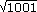x2
Introduction
=

# Testing for Primes

How can we tell if a number is prime or not? Is there a better way than dividing every number less than our number into it and seeing if it goes evenly?

Most of the time doing a lot of division is the only sure fire way to test if a number is a prime. We can reduce the number of numbers that we have to test a little with the following two theorems.

If a prime number p doesn't divide a number then none of p's multiples divide the number either.

Think about this for a minute. Say I give you a number like 21350345251. You can tell right away, by the rules at the end of Section 2.1 that 2 doesn't go into it evenly because its last digit, 1, isn't divisible by 2. But if 2 doesn't go into our number evenly then the previous theorem says that 4, 6, 8, 10, 12, ... don't go into it evenly either because they're all multiples of 2. Why? Look at this

 21350345251 = 21350345251 6 3 · 2

But because 2 doesn't go into 21350345251, it really doesn't matter what happens with the 3. So the bottom line is that, because of the theorem, we only have to test the prime numbers less than our number.

It turns out that we can do even better than that.

An integer n is composite, i.e. not prime, if and only if it's divisible by some prime number p.

This means that if I asked you to determine if 1001 was prime you'd only have to test the prime numbers less than= 31.635. Specifically, that means testing 3, 5, 7, 11, 13, 17, 19, 23, 29, and 31. Some quick work with a calculator shows that 1001 = 7 ·143 so 1001 can't be prime.

At this point you might be asking, "Where did you get that list of primes?" The honest answer is that I just went through the numbers from 2 to 31 in my head and did a quick factorization of each. If you need a larger list, we'll see a way to generate it in the next section.

### The Big Picture

I hate to be the one to break this to you but, in partical terms, there really isn't a way to decide if a really large number is prime without doing a lot of long division. Again, in practical terms, this means there's no way to factor really large numbers even using computers. By really large, I'm talking about ones with over 100 digits. Testing a number that large would require in the neighbourhood of 8 x 1047 calculations, i.e. 8 followed by 47 zeros. Even the fastest of modern computers would take in excess of 15 billion years to do them all. That's longer than the current estimate of the age of the universe!